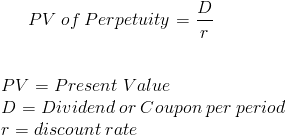# Perpetuity PaymentThe formula for the payment on a perpetuity is its present value times its rate. A perpetuity is a type of annuity where the payments continue on infinitely.

Examples of situations where a perpetuity payment formula may be used is in perpetual lease contracts, preferred stocks, consols in the UK, or any other situation where payments do not have an expiration. Preferred stocks can use this formula as dividends tend to be stable and constant. What can change is the discount rate used to calculate the value of a perpetuity. As this changes, assuming the payment stays constant, the value may change.

## Example of the Perpetuity Payment Formula

An example of the perpetuity payment formula would be to assume that an indefinite annuity is priced at \$1000 and has a rate of 10%. This can be shown as:The payment in this situation would be \$100. Keep in mind that the 10% rate may not always be explicitly stated. Stocks for companies that have zero growth are essentially similar to a perpetuity and theoretically valued the same. The 10% discount rate may be what the investor would want on a particular company with no growth. In this case, this investor could calculate what the annual dividend would need to be to match this 10% discount rate.

As a side note, risk is not calculated in this formula. Perpetuities are more theoretical than a guarantee. For example, a company with preferred stock could go under in 2 years.

## How is the Perpetuity Payment Formula derived?

The formula to calculate the payment on a perpetuity can be found by first looking at the present value of a perpetuity formula:The dividend, or payment, can be isolated by multiplying both sides by the rate. This will result in the formula at the top of the page, the present value times the rate.

New to Finance?